Area under a curve using further integration hmmm...

dfx

Posted 26 June 2005 - 08:20 PM

Say you have an equation y = 3/(x+1) and you want to find the area under the curve between the x =2 and x = 6.

So y = 3(x+1) ^-1

Now according to what we were taught in further integration, it would end up as

y = [ 3(x+1)] / 0 ...

So is there any way round this? Other than using other numerical methods such as the trapezium rule to estimate the area.

Discogirl17

Posted 26 June 2005 - 08:31 PM

You have the equation y=3/(x+1)
so y=3/x+3/1
y=3/x +3
y=3x^-1 +3
y=3+3x

no?
Nope I have no idea. Ignore that whole bit.

dfx

Posted 26 June 2005 - 08:36 PM

Nah don't think you can do that unless you use partial fractions or something?

Discogirl17

Posted 26 June 2005 - 08:37 PM

Yeh thats what I'm on about.

George

Posted 26 June 2005 - 10:04 PM

This is covered in Advanced Higher Maths, using the rule: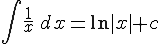So for the example you gave: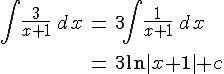Hope that doesn't look too scaryAppleCore

Posted 26 June 2005 - 10:08 PM

That does look crazzzy to me.. then again, i didnt do ADV maths lol!

dfx

Posted 26 June 2005 - 11:20 PM

Is that Mod x+1 or big brackets x+1 ?

Discogirl17

Posted 27 June 2005 - 01:14 PM

Its mod (x+1) not mod (x) +1
Is that what you were asking?

George

Posted 27 June 2005 - 02:14 PM

Yes, it's the absolute value of (x+1), since ln isn't defined for negative numbers.

dfx

Posted 27 June 2005 - 11:54 PM

erm... and how would you go about solving it?I don't understand why its an absolute value, is it cause the asymptote (presumably) never crosses the ordinate into the negative zone?

Steve

Posted 28 June 2005 - 10:27 AM

Just in case, the absolute value of a number just means that if it is negative, you make it positive, eg |-2| = 2

The reason its ln|x+1| is because the function ln only has positive real numbers in its domain. Think about the graph of y=ln(x), ie ln(x) is undefined for x0.

As an example, consider the equation: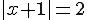Now for the equation to hold, x+1 can be -2 or 2. So x = -3 or x = 1 is the solution.

If you want to find the area under the curve of y = 1/x however, you just have to sub in the limits as usual into ln|x|. For example, the area between x = 1 and x = 3: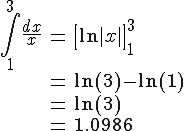So the area is 1.0986 square units

The Wedge Effect

Posted 28 June 2005 - 04:15 PM

QUOTE(Steve @ Jun 28 2005, 11:27 AM)
Just in case, the absolute value of a number just means that if it is negative, you make it positive, eg |-2| = 2

The reason its ln|x+1| is because the function ln only has positive real numbers in its domain. Think about the graph of y=ln(x), ie ln(x) is undefined for x0.

As an example, consider the equation:Now for the equation to hold, x+1 can be -2 or 2. So x = -3 or x = 1 is the solution.

If you want to find the area under the curve of y = 1/x however, you just have to sub in the limits as usual into ln|x|. For example, the area between x = 1 and x = 3:So the area is 1.0986 square units*Stares blankly, drool dripping, as if I am braindead*I'll probs be doing that stuff in uni anyway.

dfx

Posted 28 June 2005 - 11:04 PM

Hmmm.. oky doke.. thanks muchos.

Em, what about areas under y = a^x, say 2^x ? I hate learning this trapezium rule so I'm lookin for other methods lol. Thanks.George

Posted 29 June 2005 - 08:29 AM

Apparantly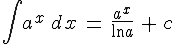I had to look that up in my calculus textbook though; it's not something I've really met yet.

dfx

Posted 29 June 2005 - 01:52 PM

You're amazing. ThanksNow for some show off to the teacher